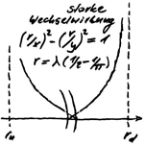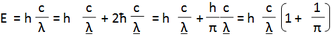# Die eine Quantentheorie

Die eine Quantentheorie
Menue in German and English - select!
Es geht um die einfachste Stringtheorie nach Art der ART,
die den Aufbau der Elementarteilchen erklärt.
Phänomene und Paradoxa lassen sich math. begründen.
Benutzt werden Falsifikation und das Noether-Theorem
mit der letzten Invarianz der Quanten.

It's about the simplest string theory in the way of the GR,
which
explains the structure of the elementary particles.
Phenomena and paradoxes can be justified mathematically.
Used are falsification and the Noether theorem with
the last invariance of the quanta.
The one quantum theory
Direkt zum SeiteninhaltDiese Seite ist
frei von Esoterik
und Quantenlogik!
Die Theoie
der Oszis
All known elementary particle models are neither perfect nor complete. Under the established quantum theory I would like to understand everything that led to the development of the Standard Model from a quantum theoretical point of view. This ranges from quantum mechanics (QM) to quantum electrodynamics (QED) to quantum chromodynamics (QCD). The QM with the measuring problem and also the QED with the radiation reaction violate the mathematical logic: Quantum logic becomes necessary! This is to be regarded as a corpse in the cellar of physics, unless the established quantum theory is clearly delineated.

The established quantum theory: His name should be Standard Quantum Theory as he is the reason for the Standard Model. I see in her the quantum theory of experimental physics, because to follow quantum processes by measuring them encounters the problem that the measuring technique itself influences the process, since the lowest level is the quantum level. The evaluation is carried out with the help of the Schrödinger equation. Only in a few cases does the underlying field theory allow results to be obtained. When interpreting them, it should not be forgotten that statistical totals are involved. In addition, quantum logic is required, because it is the only way to deal with the measurement problem without contradictions. This cannot be the one quantum theory, because it is incompatible with GRAVITATION.

The one quantum theory: It naturally includes GRAVITATION. In epistemological terms, quanta mark the lowest level, which should lead to the theory developing by itself. In terms of proof, this can be achieved by falsifying the appropriate hypothesis, which must be compatible with the general theory of relativity. The falsification is based on the statement that results from the fact that quanta lie at the lowest level of knowledge. This approach clearly gives rise to a quantum theory, without quantum logic!

Attention, since one theory does without quantum logic, but the other does not, they can be mutually contradicted!

Exactly for this reason, in this contribution value is placed on the representation of logical structures and proof techniques. The mathematical criterion, which is quantum theory, is well known and is fulfilled by the theory we are dealing with here. The elementary particle model resulting from the Noether theorem corresponds - subject to its verification - to the experimental observations and shows the geometry of the elementary particles. The quantum spook can now be justified mathematically!

The difference between established quantum theory and the one is fundamental: While one is based on the real experiment, the other uses the thought experiment. The real experiment requires mathematics, which leads to the loss of physical reality. The thought experiment leads to a variation problem whose solutions make the elementary particles real as a string model. The predictions that can be derived from this not only confirm the real experiments, but go far beyond them, resulting in new experiments!
last modification 12.04.2019
THE APPORACHBased on Einstein's light quantum hypothesis, the validity of the general theory of relativity leads to the following equation, the missing "matter quantum hypothesis" (see right):

Planck's quantum exists, but there is still no theory worthy of the name quantum theory. It is about a new beginning in quantum theory! So nobody can claim any more that the above equation is quantum theoretical nonsense. The new theory shall be created by falsification of the above equation with the following statement:

A quant is only a quant if it does not make itself dependent in any of its properties on another quant!

One could jokingly ask who is actually interested in the properties? The dilemma in which quantum theory has been stuck for over 100 years dissolves with the dilemma to which the above statement leads - the staircase joke of quantum theory. To free oneself from this new dilemma by falsification is what creates the theory, because falsification does not necessarily mean that the approach must be rejected.  In the present case, the contradiction can only be avoided under one condition at a time, so that the properties are clear.

Without further reading, every physicist should come to the same quantum theory!
last modification 09.03.2019
go to side  0  1  2  3  4  5  6  7  8  9
Zurück zum Seiteninhalt